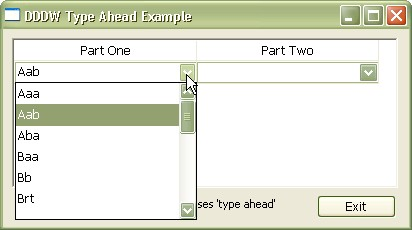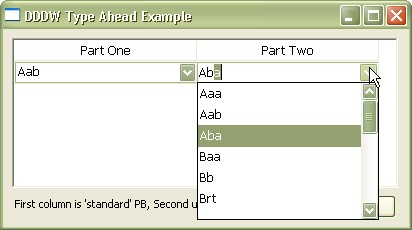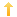## PowerBuilder – Type ahead style dropdown datawindow columns

Posted on Monday, June 6th, 2011 at 8:20 pm in

This type of functionality is one of those things which fills a gap so completely and perfectly that when you encounter some application (and almost all web pages) which doesn’t have it you groan to yourself. In the ancient past this was alternatively referred to as ‘Quicken style’ dropdowns since that application was one very common example in use. Basically this functionality is used on dropdown datawindows to allow the user to type in a series of characters which cause the control to filter the entries so that finding the desired one is quick and easy. The PFC has a service attached to the datawindow ancestor which does the same thing but here is the same functionality encapsulated into a single NVO.

Sample Application Screen Shots:Image one shows the standard PB dropdown which is basically useless outside of using the mouse pointer.Image two shows the Type Ahead interface where the highlighted row in the dropdown responds to user typing.

On the window containing the datawindow(s) you wish to have this functionality add the following:

```//getfocus
// Use this method if you have several datawindows on a window which you wish to have this type of functionality.  If not you
// could just put this code in the window postopen type event.
datawindow ldw
ldw = THIS
nvo_grid.uf_set_current_dw(ldw)

//editchanged
// map nvo event to datawindow event
CHOOSE CASE dwo.name
CASE 'pri_pro_int_id'
nvo_grid.event ue_editchanged(row, dwo, data)
END CHOOSE

//itemfocuschanged
// map nvo event to datawindow event
nvo_grid.event ue_itemfocuschanged(row, dwo)```

The NVO is set to autoinstantiate and contains the following code.

```instance variables
// dddw search as you type functionality
datawindow idw
datawindowchild idwc[]
string is_datawindow[] // names of datawindow/column combinations registered for typeahead functionality
integer     ii_currentindex
boolean		ib_performsearch=False
string		is_textprev```

Two events.

```ue_editchanged(ref long al_row, ref dwobject adwo, ref string as_data);
// mapped to editchanged event on datawindow where dddw typeahead is desired
boolean		lb_matchfound=False
integer		li_searchtextlen
long		ll_findrow
long		ll_dddw_rowcount
string		ls_dddw_displaycol
string		ls_foundtext
string		ls_findexp
string		ls_displaydata_value
string		ls_searchtext

// Check requirements.

// Confirm that the search capabilities are valid for this column.
if ib_performsearch=False or ii_currentindex <= 0 THEN return

// Get information on the column and text.
ls_searchtext = as_data
li_searchtextlen = Len (ls_searchtext)

// If the user performed a delete operation, do not perform the search.
// If the text entered is the same as the last search, do not perform another search.
If (li_searchtextlen < Len(is_textprev)) or &
(Lower (ls_searchtext) = Lower (is_textprev)) Then
// Store the previous text information.
is_textprev = ''
Return
End If

// Store the previous text information.
is_textprev = ls_searchtext

// Build the find expression to search the dddw for the text
// entered in the parent datawindow column.
ls_findexp = "Lower (Left (" + ls_dddw_displaycol + ", " + &
String (li_searchtextlen) + ")) = '" + Lower (ls_searchtext) + "'"

// Perform the Search on the dddw.
ll_dddw_rowcount = idwc[ii_currentindex].rowcount()
ll_findrow = idwc[ii_currentindex].Find (ls_findexp, 0, ll_dddw_rowcount + 1)

// Determine if a match was found on the dddw.
lb_matchfound = (ll_findrow > 0)

// Set the found text if found on the dddw.
if lb_matchfound then
// Get the text found.
ls_foundtext = idwc[ii_currentindex].GetItemString (ll_findrow, ls_dddw_displaycol)
End If

// For either dddw or ddlb, check if a match was found.
If lb_matchfound Then
// Set the text.
idw.SetText (ls_foundtext)

// Determine what to highlight or where to move the cursor..
if li_searchtextlen = len(ls_foundtext) THEN
// Move the cursor to the end
idw.SelectText (Len (ls_foundtext)+1, 0)
else
// Hightlight the portion the user has not actually typed.
idw.SelectText (li_searchtextlen + 1, Len (ls_foundtext))
end if
end if

// set index if column is in dddw array

int		li_index
string 	ls_dwcolname

ib_performsearch = False
ii_currentindex = 0
is_textprev = ''

If IsNull(al_row) or al_row <= 0 Then Return
If IsNull(idw) or Not IsValid(idw) Then Return
// Get column name. (in 'datawindow name|column name' format)
ls_dwcolname = idw.classname() + '|' + adwo.Name

// Check if column is in the search column array.
li_index = uf_find_registered_dddw_columns(ls_dwcolname)
If li_index <= 0 Then Return
// can perform search on the column
ib_performsearch = True

// Store the current index.
ii_currentindex = li_index
// Store the previous text information.
is_textprev = idw.GetText()```

Three functions

```public function integer uf_register_dddw_columns (ref datawindow adw, string as_colname);
// set up datawindowchild array
datawindowchild ldwc
integer li_rc
long	ll_ac
string ls_desc, ls_val

FOR ll_ac = 1 to Upperbound(is_datawindow)
IF is_datawindow[ll_ac] = adw.classname() + '|' + as_colname THEN
END IF
NEXT

ll_ac = upperbound(is_datawindow) + 1

is_datawindow[ll_ac] = adw.classname() + '|' + as_colname
// need allowedit property set for this functionality to work
// this must be done prior to the getchild call
ls_desc = as_colname + '.dddw.allowedit'
IF ls_val <> 'yes' THEN
ls_desc += '=yes'
END IF

// Get a reference to the DropDownDatawindow.
IF li_rc<>1 THEN RETURN -1
idwc[ll_ac] = ldwc
RETURN 1

public function integer uf_find_registered_dddw_columns (string as_dwcolname);
// finds array position of given datawindow name/column name array
integer	li_count
integer	li_i

// Get the size of the array.
li_count = upperbound(is_datawindow)

// Check for an empty array.
if li_count <= 0 THEN return 0

// Find column name in array.
for li_i=1 TO li_count
if is_datawindow[li_i] = as_dwcolname THEN
return li_i
end if
next
RETURN 0

public subroutine uf_set_current_dw (ref datawindow adw);
//called from getfocus event on datawindow	with dddw typeahead capability